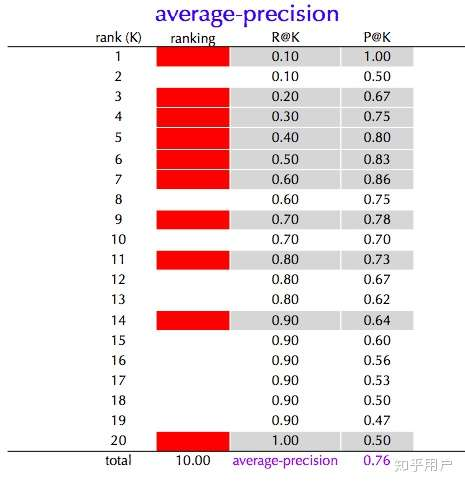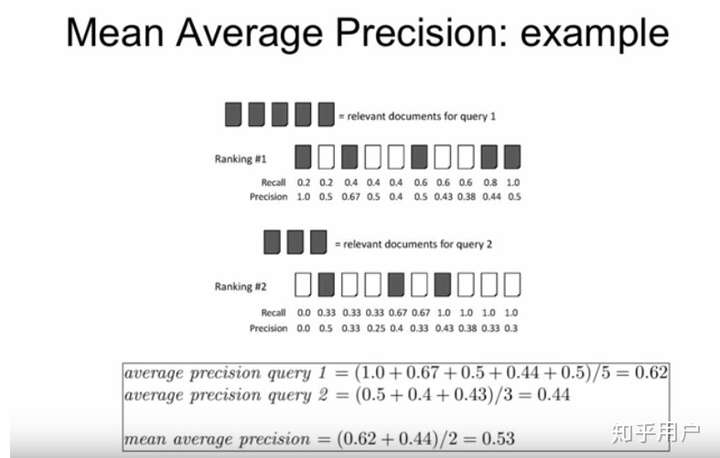# tf中tf.metrics 坑AP precision 不同的原因就是 AP 考虑了 recall 的因素，我理解的AP的概念就是同一个推荐系统内，针对不同的 recall （0.10--1.00）值记录一个对应的 precision，然后计算所有 recall 值对应 precision的平均值。上图的 AP值 即为 10个灰色部分的precision之和除以10，得到最终结果0.76。

mAP的计算，我是看到这张图，才有点理解的。labels, predictions, k, weights, metrics_collections, updates_collections, name

Github的issue找到的。

import tensorflow as tf
import numpy as np

y_true = np.array([, , , , , ]).astype(np.int64)
y_true = tf.identity(y_true)

y_pred = np.array([[0.1, 0.2, 0.6, 0.1],
[0.8, 0.05, 0.1, 0.05],
[0.3, 0.4, 0.1, 0.2],
[0.6, 0.25, 0.1, 0.05],
[0.1, 0.2, 0.6, 0.1],
[0.9, 0.0, 0.03, 0.07]]).astype(np.float32)
y_pred = tf.identity(y_pred)

_, m_ap = tf.metrics.sparse_average_precision_at_k(y_true, y_pred, 2)

sess = tf.Session()
sess.run(tf.local_variables_initializer())

stream_vars = [i for i in tf.local_variables()]
print((sess.run(stream_vars)))

tf_map = sess.run(m_ap)
print(tf_map)

tmp_rank = tf.nn.top_k(y_pred,4)
print(sess.run(tmp_rank))
1. 简单解释一下，首先y_true代表标签值（未经过one-hot）shape：(batch_size, num_labels)  ,y_pred代表预测值（logit值） ，shape：(batch_size, num_classes)
2. 其次，要注意的是tf.metrics.sparse_average_precision_at_k中会采用top_k根据不同的k值对y_pred进行排序操作 ，所以tmp_rank是为了帮助大噶理解究竟y_pred在函数中进行了怎样的转换。
3. 然后，stream_vars = [i for i in tf.local_variables()]这一行是为了帮助大噶理解 tf.metrics.sparse_average_precision_at_k创建的tf.local_varibles 实际输出值，进而可以更好地理解这个函数的用法。
4. 具体看这个例子，当k=1时，只有第一个batch的预测输出是和标签匹配的 ，所以最终输出为：1/6 = 0.166666 ；当k=2时，除了第一个batch的预测输出，第三个batch的预测输出也是和标签匹配的，所以最终输出为：(1+(1/2))/6 = 0.25
posted @ 2019-06-11 20:12 胡里糊涂 阅读(...) 评论(...) 编辑 收藏